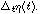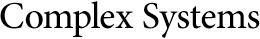## Complex Systems: Errata and Changes, Volume 1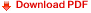Bruce M. Boghosian

C. David Levermore

Daniel G. Maeder

Kristian Lindgren

#### Abstract

All known errata and changes are given below for articles published in Complex Systems, Volume 1, 1987.

Bruce M. Boghosian and C. David Levermore, "A Cellular Automaton for Burgers' Equation," pages 17-29

The two columns of figures on page 27 are transposed; they are labelled correctly.

The words "Below we plot" on page 28 refer to the plots on page 27.

Daniel G. Maeder, "The Free Energy Concept in Celluar Automaton Models of Solid-Solid Phase Transitions," pages 131-143

The caption for figure 2 should be replaced by the following:

Printout of a hypothetical two-dimensional cellular automaton model for a shape memory alloy, at a temperature producing about one-third of martensite in equilibrium with two thirds of austenite. The pattern resembles some enlarged details of figure 1c where the variants A, B, C, D show up as different gray shades. For better contrast, austentic cells have been represented by colons rather than by a letter. At high temperature, each martensitic plate must shrink into a single permanent "germ" cell of the respective variant. A realistic model should contain very few germs (i.e. crystal defects) per 1000 cells.

Kristian Lindgren, "Correlations and Random Information in Cellular Automata," pages 529-543

Equation (2.11) should be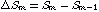In section 3, "Algorithmic information theory," the notation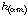should be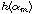,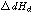should be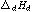,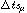should be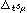. In equation (4.1),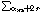should be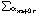.

Equation (4.5) should be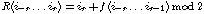The second part of equation (4.7) should be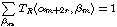The second half of equation (4.14) is missing a right parenthesis before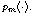In section 4,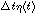should be